# RS Aggarwal Class 10 Solutions Chapter 3 - Linear Equations In Two Variables - Ex 3C(3.3)

## RS Aggarwal Class 10 Chapter 3 - Linear Equations In Two Variables - Ex 3C(3.3) Solutions Free PDF

For Class 10 students it is important to start the preparation early and learn new concepts properly to develop a strong foundation for the future. These RS Aggarwal Class 10 Solutions will help students in clearing their concepts and doubts related to the exercise questions. It is considered a highly beneficial resource for students preparing well for the exams. For the benefit of the students, we at BYJU’S have provided highly effective solutions of RS Aggarwal Class 10 solutions to help them to master the difficult maths concept very easily.

Prepared by subject experts the RS Aggarwal Solutions Class 10 Chapter 3 – Linear Equations In Two Variables strictly follow the syllabus of CBSE. All the solutions comprise of step-by-step explanations for important questions given in Class 10 RS Aggarwal textbook. It also acts as an alternative to supplement a student’s exam preparation and to help them score good marks.

## Download PDF of RS Aggarwal Class 10 Solutions Chapter 3 – Linear Equations In Two Variables – Ex 3C (3.3)

Question 1: On a graph paper, draw a horizontal line X’OX and a vertical line YoY’ as the x-axis and the y-axis respectively.

5x – y = 7, x – y + 1 = 0

Solution:

Given equations are 5x – y = 7 and x – y + 1 = 0

Graph of 5x – y = 7:

5x – y = 7 $\Rightarrow y = 5x – 7$

Putting x = 0, we get y = -7

Putting x = 1, we get y = -2

Putting x = 2, we get y = 3

Hence, the table is,

x012y-7-23

Plot the points A(0, 7), B(1, -2) and C(2, 3) on the graph paper. Join AB and BC to get the graph line AC. Extend it both ways.

Thus, line AC is the graph of 5x – y = 7.

Graph of x – y + 1 = 0:

x – y + 1 = 0 $\Rightarrow y =x+ 1$

Putting x = 0, we get y = 1

Putting x = 1, we get y = 2

Putting x = 2, we get y = 3

Hence, the table is,

x012y123

Now, on the same graph paper as above plot the points P(0, 1) and Q(1, 2). The point C(2, 3) has already been plotted. Join PA.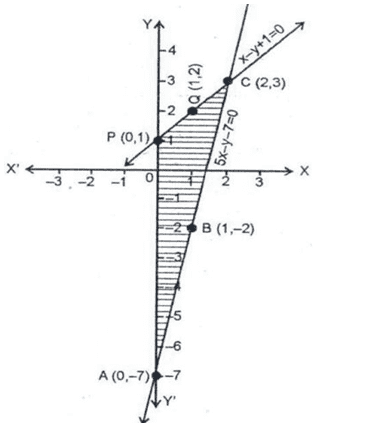Thus, line PA is the graph of 3x + 2y – 12 = 0. The two graph lines intersect at C(2, 3).

Hence, x = 2, y = 3 is the solution of the given system of equations.

Clearly, the vertices of $\Delta APC$ formed by these lines and the y-axis are A(0, 7), P(0, 1) and C(2, 3).

Consider the triangle $\Delta APC$ :

Height of the triangle = 2 units and base(AP) = 8 units

Area of the triangle $\Delta APC$:

$Area = \frac{1}{2} \times Base \times height = \frac{1}{2} \times 8 \times 2$ sq. units

Area of $\Delta APC$ = 8 sq. units

Question 2: On a graph paper, draw a horizontal line X’OX and a vertical line YoY’ as the x-axis and the y-axis respectively.

x – 2y = 2, 4x – 2y = 5

Solution:

Given equations are x – 2y = 2 and 4x – 2y = 5

Graph of x – 2y = 2:

x – 2y = 2 $\Rightarrow y = \frac{x – 2}{2}$

Putting x = 0, we get y = -1

Putting x = 1, we get y = -0.5

Putting x = 2, we get y = 0

Hence, the table is,

x012y-1-0.50

Plot the points A(0, -1), B(2, 0) and C(1, -0.5) on the graph paper. Join AC and AB to get the graph line AB. Extend it both ways.

Thus, line AB is the graph of x – 2y = 2.

Graph of 4x – 2y = 5:

4x – 2y = 5 $\Rightarrow y = \frac{4x – 5}{2}$

Putting x = 0, we get y = -2.5

Putting x = 1, we get y = -0.5

Putting x = 2, we get y = -1.5

Hence, the table is,

x012y-2.5-0.5-1.5

Now, on the same graph paper as above plot the points P(0, -0.25) and Q(2, -1.5). The point C(1, -0.5) has already been plotted. Join PC and CQ to get PQ.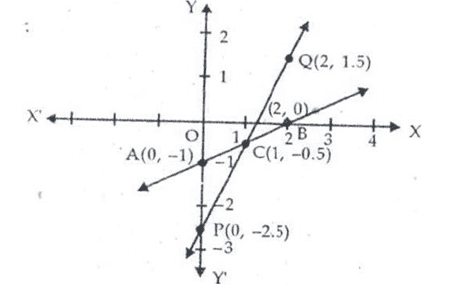Thus, line PA is the graph of 4x – 2y = 5. The two graph lines intersect at (1, -0.5).

The given system of equations is consistent.

Question 3: On a graph paper, draw a horizontal line X’OX and a vertical line YOY’ as the x-axis and the y-axis respectively.

2x + 3y = 4, 4x + 6y = 12

Solution:

Given equations are 2x + 3y = 4 and 4x + 6y = 12

Graph of 2x + 3y = 4:

2x + 3y = 4 $\Rightarrow y = \frac{-2x + 4}{3}$

Putting x = 2, we get y = 2

Putting x = -1, we get y = 2

Putting x = -4, we get y = 4

Hence, the table is,

x2-1-4y024

Plot the points A(2, 0), B(-1, 2) and C(-4, 4) on the graph paper. Join AB and BC to get the graph line AC. Extend it both ways.

Thus, line AC is the graph of 2x + 3y = 4.

Graph of 4x + 6y = 12:

4x + 6y = 12 $\Rightarrow y = \frac{-4x + 12}{6}$

Putting x = 3, we get y = 0

Putting x = 0, we get y = 2

Putting x = 6, we get y = -2

Hence, the table is,

x306y02-2

Now, on the same graph paper as above plot the points P(3, 0) and Q(0, 2) and R(6, -2). Join PQ and PR to get QR.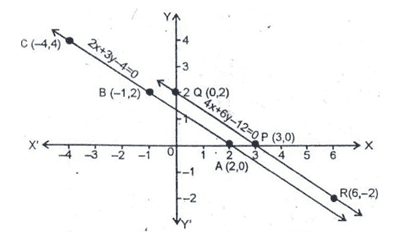Thus, line QR is the graph of 4x + 6y = 12.

It is not clear from the graph that two graph lines are parallel and do not intersect when produced.

Hence, the given system of equation is consistent.

Question 4: On a graph paper, draw a horizontal line X’OX and a vertical line YOY’ as the x-axis and the y-axis respectively.

2y – x = 9, 4y – 2x = 20

Solution:

Given equations are 2y – x = 9 and 4y – 2x = 20

Graph of 2y – x = 9:

2y – x = 9 $\Rightarrow y = \frac{x + 9}{2}$

Putting x = 1, we get y = 5

Putting x = -1, we get y = 4

Putting x = -3, we get y = 3

Hence, the table is,

x1-1-3y543

Plot the points A(1, 5), B(-1, 4) and C(-3, 3) on the graph paper. Join AB and BC to get the graph line AC. Extend it both ways.

Thus, line AC is the graph of 2y – x = 9.

Graph of 4y – 2x = 20:

4y – 2x = 20 $\Rightarrow y = \frac{2x + 20}{4}$

Putting x = 0, we get y = 5

Putting x = 2, we get y = 6

Putting x = -2, we get y = 4

Hence, the table is,

x02-2y564

Now, on the same graph paper as above plot the points P(0, 5) and Q(2, 6) and R(-2, 4). Join PQ and PR to get QR.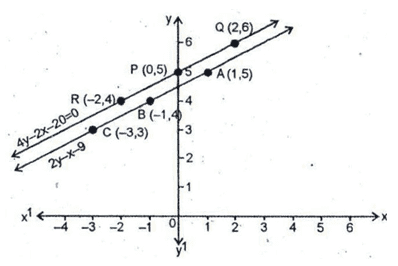Thus, line QR is the graph of 4y – 2x = 20.

It is not clear from the graph that two graph lines are parallel and do not intersect when produced.

Hence, the given system of equation is consistent.

Question 5: On a graph paper, draw a horizontal line X’OX and a vertical line YOY’ as the x-axis and the y-axis respectively.

3x – y = 5, 6x – 2y = 10

Solution:

Given equations are 3x – y = 5 and 6x – 2y = 10

Graph of 3x – y = 5:

3x – y = 5 $\Rightarrow y = 3x – 5$

Putting x = 1, we get y = -2

Putting x = 0, we get y = -5

Putting x = 2, we get y = 1

Hence, the table is:

x102y-2-51

Plot the points A(1, -2), B(0, -5) and C(2, 1) on the graph paper. Join AB and AC to get the graph line BC. Extend it both ways.

Thus, line BC is the graph of 3x – y = 5.

Graph of 6x – 2y = 10:

6x – 2y = 10 $\Rightarrow y = \frac{6x – 10}{2}$

Putting x = 0, we get y = -5

Putting x = 1, we get y = -2

Putting x = 2, we get y = 1

Hence, the table is:

x012y-5-21

These points are same as obtained above: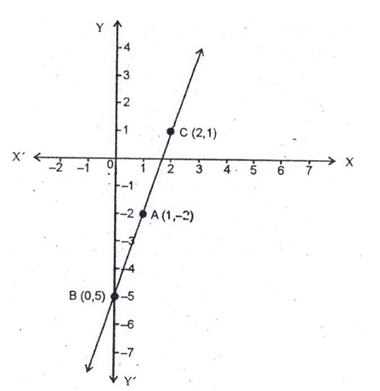From the graph, it is clear that these two lines coincide. Both equations represent same graph. Hence, these lines have infinitely many solutions.

Question 6: On a graph paper, draw a horizontal line X’OX and a vertical line YOY’ as the x-axis and the y-axis respectively.

2x + y = 6, 6x + 3y = 18

Solution:

Given equations are 2x + y = 6 and 6x + 3y = 18

Graph of 2x + y = 6:

2x + y = 6 $\Rightarrow y = -2x + 6$

Putting x = 3, we get y = 0

Putting x = 1, we get y = 4

Putting x = 2, we get y = 2

Hence, the table is,

x312y042

Plot the points A(3, 0), B(1, 4) and C(2, 2) on the graph paper. Join AC and BC to get the graph line of equation 2x + y = 6.

Graph of 6x + 3y = 18:

6x + 3y = 18 $\Rightarrow y = \frac{-6x + 18}{3}$

Putting x = 0, we get y = -5

Putting x = 1, we get y = -2

Putting x = 2, we get y = 1

Hence, the table is:

x312y042

These points are same as obtained above: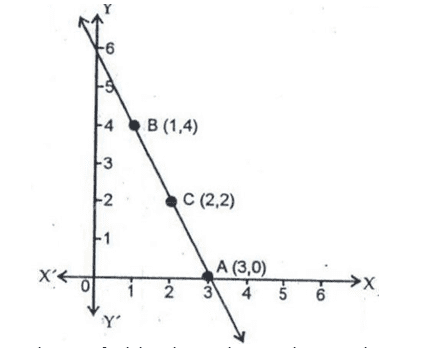Thus, we find that the two line graphs coincide. Hence, the given system of equations has infinitely many solutions.

Solve for x and y

Question 7: x + y = 8, 2x – 3y = 1

Solution:

The given equations are.

x + y = 8 …..(1)

2x – 3y = 1 …..(2)

Multiplying (1) by 3 and (2) by 1, we get,

3x + 3y = 24 …..(3)

2x – 3y = 1 …..(4)

Adding (3) and (4), we get

$5x \, = \, 25 \Rightarrow x \, = \, \frac{25}{5} \Rightarrow x \, = \, 5$

Substituting x = 5 in (1), we get

5 + y = 8 $\Rightarrow$ y = 8 – 5 = 3

∴ x = 5 and y = 3

Question 8: x + y = 3, 4x – 3y = 26

Solution:

The given equations are.

x + y = 3 …..(1)

4x – 3y = 26 …..(2)

Multiplying (1) by 3 and (2) by 1, we get,

3x + 3y = 9 …..(3)

4x – 3y = 26 …..(4)

Adding (3) and (4), we get

7x = 35 $\Rightarrow$ x = 5

Substituting x = 5 in (1), we get

5 + y = 3 $\Rightarrow$ y = 3 – 5 = -2

∴ x = 5 and y = -2

Question 9: x- y = 3, 3x – 2y

Solution:

The given equations are.

x- y = 3 …..(1)

3x – 2y = 10 …..(2)

Multiplying (1) by 2 and (2) by 1, we get,

x – y = 3 …..(3)

3x – 2y = 10 …..(4)

Subtracting (3) from (4), we get

x = 4

Substituting x = 4 in (1), we get

4 – y = 3 $\Rightarrow$ y = 4 – 3 = 1

∴ x = 4 and y = 3

Question 10: 2x + 3y = 0, 3x + 4y = 5

Solution:

The given equations are.

2x + 3y = 0 …..(1)

3x + 4y = 5 …..(2)

Multiplying (1) by 4 and (2) by 3, we get,

8x + 12y = 0 …..(3)

9x + 12y = 15 …..(4)

Subtracting (3) from (4), we get

x = 15

Substituting x = 15 in (1), we get

(2)(15) + 3y = 0 $\Rightarrow$ 3y = 0 – 30

3y = -30 or y = -10

∴ x = 15 and y = -10

Question 11: 2x – 3y = 13, 7x – 2y = 20

Solution:

The given equations are.

2x – 3y = 13 …..(1)

7x – 2y = 20 …..(2)

Multiplying (1) by 2 and (2) by 3, we get,

4x – 6y = 26 …..(3)

21x – 6y = 60 …..(4)

Subtracting (3) from (4), we get

17x = 34 $\Rightarrow$ x = 2

Substituting x = 2 in (1), we get

(2)(2) – 3y = 13 $\Rightarrow$ 4 – 3y = 13

-3y = 13 – 4 or -3y = 9 or y = -3

∴ x = 2 and y = -3

### Key Features of RS Aggarwal Class 10 Solutions Chapter 3 – Linear Equations In Two Variables – Ex 3C (3.3)

• Practice these solutions to boost up the confidence level.
• It will improve problem-solving skills, speed and accuracy.
• It provides different types of questions from each and every topic
• These solutions are the best resource material for revision purpose.

#### Practise This Question

The components of the ecosystem are: